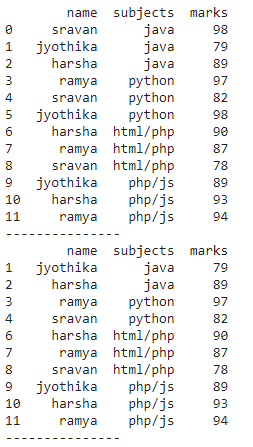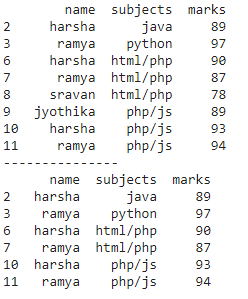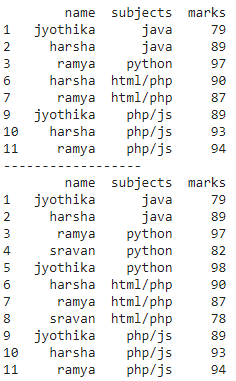Open in App
Not now

# How to Drop Rows that Contain a Specific Value in Pandas?

• Last Updated : 24 Jan, 2023

In this article, we will discuss how to drop rows that contain a specific value in Pandas. Dropping rows means removing values from the dataframe we can drop the specific value by using conditional or relational operators.

## Method 1: Drop the specific value by using Operators

We can use the column_name function along with the operator to drop the specific value.

Syntax: dataframe[dataframe.column_name operator value]

where

• dataframe is the input dataframe
• column_name is the value of that column to be dropped
• operator is the relational operator
• value is the specific value to be dropped from the particular column

## Python3

 `# import pandas module` `import` `pandas as pd`   `# create dataframe with 4 columns` `data ``=` `pd.DataFrame({`   `    ``"name"``: [``'sravan'``, ``'jyothika'``, ``'harsha'``, ``'ramya'``,` `             ``'sravan'``, ``'jyothika'``, ``'harsha'``, ``'ramya'``,` `             ``'sravan'``, ``'jyothika'``, ``'harsha'``, ``'ramya'``],` `    ``"subjects"``: [``'java'``, ``'java'``, ``'java'``, ``'python'``,` `                 ``'python'``, ``'python'``, ``'html/php'``, ``'html/php'``,` `                 ``'html/php'``, ``'php/js'``, ``'php/js'``, ``'php/js'``],` `    ``"marks"``: [``98``, ``79``, ``89``, ``97``, ``82``, ``98``, ``90``,` `              ``87``, ``78``, ``89``, ``93``, ``94``]` `})`   `# display` `print``(data)`   `print``(``"---------------"``)`   `# drop rows where value is 98` `# by using not equal operator` `print``(data[data.marks !``=` `98``])`   `print``(``"---------------"``)`

Output:## Method 2: Drop Rows that Contain Values in a List

By using this method we can drop multiple values present in the list, we are using isin() operator. This operator is used to check whether the given value is present in the list or not

Syntax: dataframe[dataframe.column_name.isin(list_of_values) == False]

where

• dataframe is the input dataframe
• column_name is to remove values in this column
• list_of_values is the specific values to be removed

## Python3

 `# import pandas module` `import` `pandas as pd`   `# create dataframe with 4 columns` `data ``=` `pd.DataFrame({`   `    ``"name"``: [``'sravan'``, ``'jyothika'``, ``'harsha'``, ``'ramya'``,` `             ``'sravan'``, ``'jyothika'``, ``'harsha'``, ``'ramya'``, ` `             ``'sravan'``, ``'jyothika'``, ``'harsha'``, ``'ramya'``],` `    ``"subjects"``: [``'java'``, ``'java'``, ``'java'``, ``'python'``, ` `                 ``'python'``, ``'python'``, ``'html/php'``, ` `                 ``'html/php'``, ``'html/php'``, ``'php/js'``, ` `                 ``'php/js'``, ``'php/js'``],` `    ``"marks"``: [``98``, ``79``, ``89``, ``97``, ``82``, ``98``, ``90``, ``87``,` `              ``78``, ``89``, ``93``, ``94``]` `})`   `# consider the list` `list1 ``=` `[``98``, ``82``, ``79``]`   `# drop rows from above list` `print``(data[data.marks.isin(list1) ``=``=` `False``])`   `print``(``"---------------"``)`   `list2 ``=` `[``'sravan'``, ``'jyothika'``]` `# drop rows from above list` `print``(data[data.name.isin(list2) ``=``=` `False``])`

Output:## Method 3: Drop rows that contain specific values in multiple columns

We can drop specific values from multiple columns by using relational operators.

Syntax: dataframe[(dataframe.column_name operator value ) relational_operator (dataframe.column_name operator value )]

where

• dataframe is the input dataframe
• column_name is the column
• operator is the relational operator

## Python3

 `# import pandas module` `import` `pandas as pd`   `# create dataframe with 4 columns` `data ``=` `pd.DataFrame({`   `    ``"name"``: [``'sravan'``, ``'jyothika'``, ``'harsha'``, ``'ramya'``,` `             ``'sravan'``, ``'jyothika'``, ``'harsha'``, ``'ramya'``,` `             ``'sravan'``, ``'jyothika'``, ``'harsha'``, ``'ramya'``],` `    ``"subjects"``: [``'java'``, ``'java'``, ``'java'``, ``'python'``,` `                 ``'python'``, ``'python'``, ``'html/php'``, ` `                 ``'html/php'``, ``'html/php'``, ``'php/js'``, ` `                 ``'php/js'``, ``'php/js'``],` `    ``"marks"``: [``98``, ``79``, ``89``, ``97``, ``82``, ``98``, ``90``,` `              ``87``, ``78``, ``89``, ``93``, ``94``]` `})`   `# drop specific values` `# where marks is 98 and name is sravan` `print``(data[(data.marks !``=` `98``) & (data.name !``=` `'sravan'``)])`   `print``(``"------------------"``)`   `# drop specific values` `# where marks is 98 or name is sravan` `print``(data[(data.marks !``=` `98``) | (data.name !``=` `'sravan'``)])`

Output:My Personal Notes arrow_drop_up
Related Articles